# Texas Go Math Grade 2 Lesson 12.2 Answer Key Describe Multiplication

Refer to our Texas Go Math Grade 2 Answer Key Pdf to score good marks in the exams. Test yourself by practicing the problems from Texas Go Math Grade 2 Lesson 12.2 Answer Key Describe Multiplication.

## Texas Go Math Grade 2 Lesson 12.2 Answer Key Describe Multiplication

Explore

Use counters to model the problem. Then draw a picture of your model. Write o number sentence for it.Robby has _________ DVDs.

FOR THE TEACHER • Read the problem to children. Then have them model the problem using counters and write a number sentence for it. Robby has 5 boxes of DVDs. Each box holds 7 DVDs. How many DVDs does Robby have?
Robby has 35 DVDs.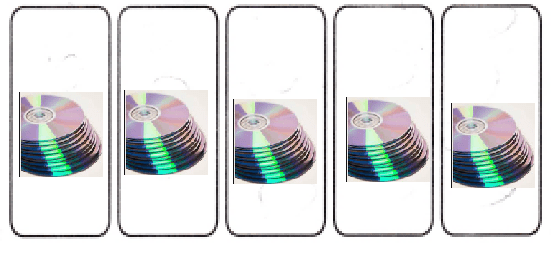7 x 5 = 35
Explanation:
Robby has 5 boxes of DVDs.
Each box holds 7 DVDs.
5 x 7 = 35
35 DVDs that Robby have

Math Talk
Mathematical Processes

Describe the groups that you drew.
Robby has 5 boxes of DVDs.
Each box holds 7 DVDs.
5 x 7 = 35
35 DVDs that Robby have

Model and Draw

When you multiply, you join equal groups. Use the pictures to describe equal groups.Explanation:
15 ÷ 3 = 5
12 ÷ 4 = 3
15 muffins shared among 3 trays
12 balls shared among 4 boxes

Share and Show

Use the pictures to describe equal groups.

Question 1.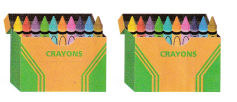There are _________ boxes of crayons.
Each ___________ has ___________.
So. there are ___________ in all.
There are 2 boxes of crayons.
Each box has 12.
So. there are 24 in all.
Explanation:
2 x 12 = 24
With the help of images found the sum

Question 2.There are ___________ bicycles.
Each ___________ has ___________.
So, there are ___________ in all.
There are 5 bicycles.
Each friend has 1 cycle.
So, there are 5 in all.
Explanation:
5 x 1 = 5
With the help of images found the sum

Problem Solving

Use the pictures to describe equal groups.

Question 3.There are ____________ stacks of books.
Each stack ___________.
So, ___________.
There are 5 stacks of books.
Each stack 3 .
So, 15 books.
Explanation:
3 x 5 = 15
With the help of images found the sum

Question 4.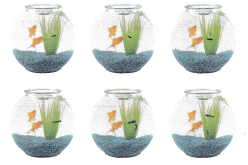There are ____________ fish bowls.
Each bowl ____________.
So, ____________.
There are 6 fish bowls.
Each bowl 2 fishes.
So, 12 fishes in all.
Explanation:
6 x 2 = 12
With the help of images found the sum

Question 5.
H.O.T. Multi-Step Use the pictures to describe the equal groups. Then write the total number of legs.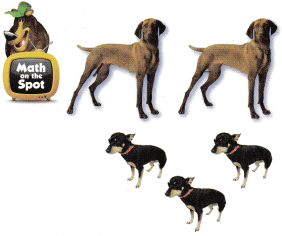5 x 4 = 20
Explanation:
5 dogs with 4 legs
5 x 4 = 20
With the help of images found the sum

Question 6.
H.O.T. Caleb uses 3 toothpicks to make a triangle. He makes 3 triangles. How many toothpicks does he use?
__________ toothpicks
9 toothpicks
Explanation:
Caleb uses 3 toothpicks to make a triangle.
He makes 3 triangles.
3 x 3 = 9
9 toothpicks that he use

Question 7.
Analyze One egg carton can hold 12 eggs. How many eggs will fit in 2 cartons?
(A) 24
(B) 12
(C) 28
Explanation:
One egg carton can hold 12 eggs.
2 x 12 = 24
24 eggs will fit in 2 cartons

Question 8.
Use the pictures to describe equal groups.There are ________ bowls of apples.
Each bowl __________________
So, __________________.
There are 4 bowls of apples.
Each bowl has 3 apples
So, 12 apples in all.
Explanation:
4 x 3 = 12
With the help of images found the sum

Question 9.
TEXAS Test Prep Tim has 5 cards. He puts 3 stickers on each card. How many stickers does he use?
(A) 8
(B) 15
(C) 10
Explanation:
Tim has 5 cards.
He puts 3 stickers on each card.
5 x 3 = 15
15 stickers that he use

TAKE HOME ACTIVITY • Ask your child to show how he or she solved an exercise in the lesson.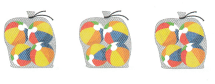There are _________ bags of beach balls.
Each bag has __________________ beach balls.
So, there are __________________ balls in all.
There are 3 bags of beach balls.
Each bag has  4 beach balls.
So, there are 12 balls in all.
Explanation:
3 x 4 = 12
With the help of images found the sum

### Texas Go Math Grade 2 Lesson 12.2 Homework and Practice Answer Key

Use the pictures to describe equal groups.

Question 1.There are _________ bags of beach balls.
Each bag has __________________ beach balls.
So, there are __________________ balls in all.
There are 3 bags of beach balls.
Each bag has  4 beach balls.
So, there are 12 balls in all.
Explanation:
3 x 4 = 12
With the help of images found the sum

Question 2.There are __________ starfish.
Each starfish ____________________ .
So, __________________.
There are 6 starfish.
Each starfish 5 fingers.
So, 30 fingers.
Explanation:
6 x 5 = 30 fingers
With the help of images found the sum

Question 3.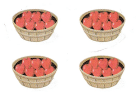There are _________ baskets of apples.
So, __________________.
There are 4 baskets of apples.
So, 32 apples
Explanation:
4 x 8 = 32
With the help of images found the sum

Problem Solving

Solve. Write or draw to explain.

Question 4.
Multi-Step Use the picture to describe the equal groups of toy animals. Then write the total number of legs.2 cubs
and 2 loins
2 + 2 = 4
4 x 4 = 16
Explanation:
the total number of legs = 16
With the help of images found the sum

Lesson Check

Question 5.
Ken has 3 boxes of sandwiches. Each box has 6 sandwiches. How many sandwiches does Ken have?
(A) 9
(B) 18
(C) 15
Explanation:
Ken has 3 boxes of sandwiches.
Each box has 6 sandwiches.
3 x 6 = 18
18 sandwiches that Ken have

Question 6.
Three girls are making puppets. Each puppet takes I hour to make. How many puppets will the girls make in 3 hours?
(A) 3
(B) 9
(C) 6
Explanation:
Three girls are making puppets.
Each puppet takes I hour to make.
3 x 3 = 9
9 puppets will the girls make in 3 hours

Question 7.
Carl has 4 stacks of 5 pennies. How many pennies does he have in all?
(A) 20
(B) 50
(C) 100
Explanation:
Carl has 4 stacks of 5 pennies.
4 x 5 = 20
20 pennies that he have in all

Question 8.
There are 6 pigs in a pen. Each pig gets a pail of food twice a day. How many pails of food are used each day?
(A) 14
(B) 8
(C) 12